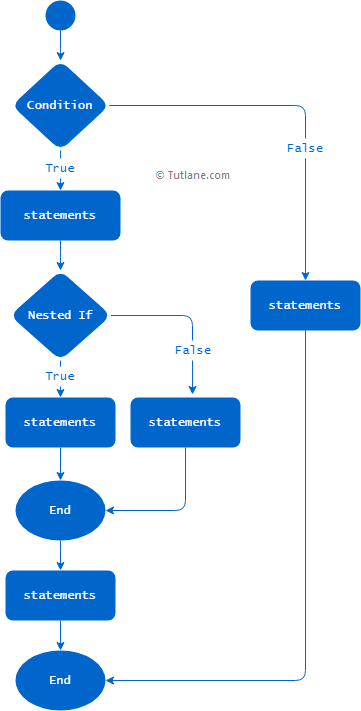# Python Nested If Else

If you create if-else statements inside other if-else statements, we will call them nested if-else statements in python. The nested if-else statements are useful when you want to execute the block of code/statements inside other if-else statements.

## Nested If Else Statement Syntax

Following is the syntax of defining nested if-else statement in python by adding an if-else statement inside another if-else statement.

if boolean_expression:
if boolean_expression:
statement(s)
else:
statement(s)
else:
statement(s)

If you observe the above nested if-else statement syntax, we created an if-else statement inside another if-else statement.

As discussed in python if and python if-else statements, you need to follow the indentation while creating the nested if-else block statements otherwise, you will get build errors (`IndentationError`).

To know more about specifying the indentation in python, refer to Python basic syntaxes.

## Python Nested If Else Flow Chart

Following is the flow chart diagram of the nested if-else statements process flow in python.If you observe the above nested if-else statement flow chart diagram, we added an if-else statement inside another if-else statement to execute the block of statements based on our requirements.

## Nested If Else Statement Example

Following is the example of a nested if-else statement in python to execute the required block of statements based on the defined Boolean expression.

x = 30
y = 10
if x >= y:
print("x is greater than or equals to y")
if x == y:
print("x is equals to y")
else:
print("x is greater than y")
else:
print("x is less than y")

If you observe the above example, we defined an if-else statement inside another if-else statement with proper indentation to execute the respective block of statements.

When you execute the above python program, you will get the result as shown below.

x is greater than or equals to y
x is greater than y

This is how you can create nested if-else statements in python to execute the block of statements based on your requirements.